Previous: 6.1.1 Wave Mechanics Up: 6.1.1 Wave Mechanics Next: 6.1.1.2 Von Neumann Equation

### 6.1.1.1 Schrödinger Equation

The time-dependent single-particle Schrödinger equation is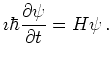(6.1)

Here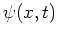is the complex wave function. The Hamiltonian operator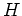is a Hermitian (self-adjoint) linear operator acting on the state space. The Hamiltonian describes the total energy of the system.

For a particle with potential energy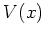the Hamiltonian is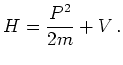(6.2)

If the effective mass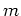is space dependent we choose the operator ordering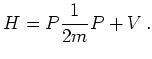(6.3)

to get a self-adjoint Hamiltonian. In all our applications the effective mass is piecewise constant.

Position and momentum operators obey the canonical commutation relations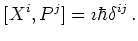(6.4)

In one-dimensional position space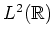the position operator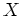is given by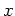(i.e., multiplication of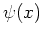by). The momentum operator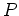is then given by. With this the transient Schrödinger equation for the electron wave functionon the real line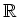reads (with constant mass)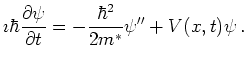(6.5)

In the so called time-independent case the wave function is of the form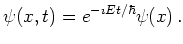(6.6)

As time progresses, the state vectors change only by a complex phase and Schrödinger's equation becomes an eigenvalue equation for the Hamiltonian,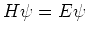(6.7)

or with 6.2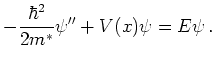(6.8)

The inner product in the Hilbert space determines the probabilistic structure. Observables are represented by self-adjoint operators. The inner product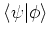of two vectors is given by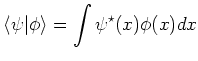(6.9)

where we used Dirac's bra-ket'' notation. The eigenvectors of the position operator are denoted by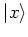, we write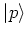for the eigenvectors of the momentum operator. The wave functionsand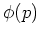are then recovered as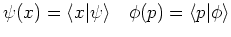(6.10)

for abstract wave vectors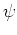,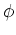. The relation beween space and momentum representation is a Fourier transformation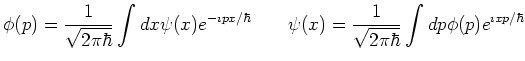Observables are repesented by self-adjoint operators. In the Dirac notation the expectation value of an observable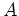given in stateis denoted by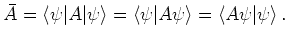(6.11)

Note that for non self-adjoint operators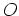the notation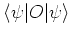is ambiguous.